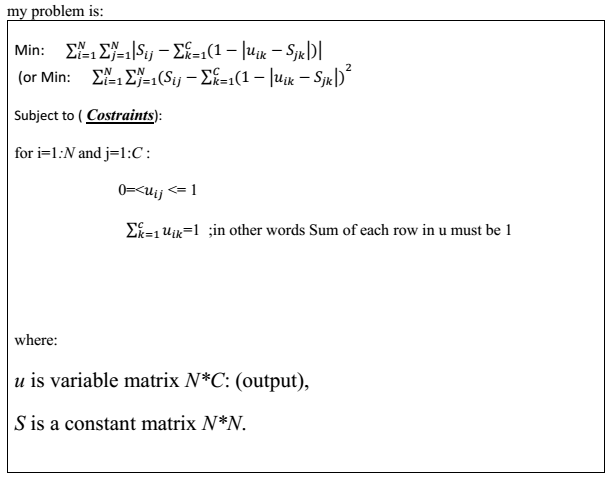# Is convex or not convex problem?

I encountered a problem, which is was attached.

Is it a convex problem? (how prove?)

If it is not convex, how I convert it to a convex problem?On this forum, you should be telling us a problem is convex and supplying the proof. Not asking whether the problem is convex. If the problem ix convex, forum readers might be able to help determine whether it can be entered in CVX and provide tips on how to do so.

I will presume the models (specifically, objective functions) are non-convex unless you show otherwise.

Thank
I enters the problem in CVX, but CVX says iit is not convex.
how to convert (approximate) it to a convex problem?
with regards

We have no idea why you want to solve this problem, and therefore which approximate problem which is convex would be of interest to you. So formulating an alternative problem is your responsibility, and is outside the scope of this forum.

The constraints are linear, so those are convex.